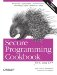# Randomly Shuffling Data

Problem

You have a sequence of data, and you need to jumble it into some random order.

Solution

Use the random_shuffle standard algorithm, defined in . random_shuffle takes two random-access iterators, and (optionally) a random-number generation functor, and rearranges the elements in the range at random. Example 7-3 shows how to do this.

Example 7-3. Shuffling a sequence at random

```#include
#include
#include
#include
#include "utils.h" // For printContainer( ): see 7.10

using namespace std;

int main( ) {

vector v;
back_insert_iterator > p =
back_inserter(v);

for (int i = 0; i < 10; ++i)
*p = i;

printContainer(v, true);

random_shuffle(v.begin( ), v.end( ));

printContainer(v, true);
}```

Your output might look like this:

```-----
0 1 2 3 4 5 6 7 8 9
-----
8 1 9 2 0 5 7 3 4 6```

Discussion

random_shuffle is intuitive to use. Give it a range, and it will shuffle the range at random. There are two versions, and their prototypes look like this:

```void random_shuffle(RndIter first, RndIter last);
void random_shuffle(RndIter first, RndIter last, RandFunc& rand);```

In the first version, the "random" is using an implementation-specific random-number generation function, which should be sufficient for most of your needs. If it isn'tperhaps you want a nonuniform distribution, e.g., Gaussianyou can write your own and supply that instead using the second version.

Your random-number generator must be a functor that a single argument and returns a single value, both of which are convertible to iterator_traits::difference_type. In most cases, an integer will do. For example, here's my knock-off random-number generator:

```struct RanNumGenFtor {
size_t operator( )(size_t n) const {
return(rand( ) % n);
}
} rnd;

random_shuffle(v.begin( ), v.end( ), rnd);```

The applications to random_shuffle are limited to sequences that provide random-access iterators (strings, vectors, and deques), arrays, or your custom containers that do the same. You can't randomly shuffle an associative container because its contents are stored in sorted order. In fact, you can't use any algorithm that modifies its range (often referred to as a mutating algorithm) on an associative container.Secure Programming Cookbook for C and C++: Recipes for Cryptography, Authentication, Input Validation & More
ISBN: 0596003943
EAN: 2147483647
Year: 2006
Pages: 241